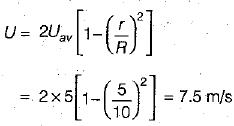Courses

# Laminar Flow In Pipes - 2

## 6 Questions MCQ Test Mock Test Series for Civil Engineering (CE) GATE 2020 | Laminar Flow In Pipes - 2

Description
This mock test of Laminar Flow In Pipes - 2 for Civil Engineering (CE) helps you for every Civil Engineering (CE) entrance exam. This contains 6 Multiple Choice Questions for Civil Engineering (CE) Laminar Flow In Pipes - 2 (mcq) to study with solutions a complete question bank. The solved questions answers in this Laminar Flow In Pipes - 2 quiz give you a good mix of easy questions and tough questions. Civil Engineering (CE) students definitely take this Laminar Flow In Pipes - 2 exercise for a better result in the exam. You can find other Laminar Flow In Pipes - 2 extra questions, long questions & short questions for Civil Engineering (CE) on EduRev as well by searching above.
QUESTION: 1

### A fluid of kinematic viscosity 0.4 cm2/sec flows through a 8 cm diameter pipe. The maximum velocity for laminar flow will be

Solution:

Maximum Reynold's number for laminar flow = 2000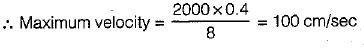QUESTION: 2

Solution:
QUESTION: 3

### In a two-dimensional flow of a viscous fluid couette flow is defined for

Solution:

Coutte flow is characterized as flow of very low value of Reynolds number between two parallel plate, one is fixed and other is movable.

QUESTION: 4

Consider the following statements regarding the laminar flow through a circular pipe:
1. The friction factor is constant.
2. Thp friction factor dspends Upon the pipe roughness.
3. The friction factor varies inversely with the Reynolds number of flow.
4. The velocity distribution is parabolic.
5. The pressure drop varies directly with the mean velocity.
Of these statements:

Solution:

For laminar flow th rough circular pipe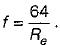It is neither constant, and does not depend on pipe roughness.
As head loss varies directly with mean velocity so pressure drop also.

QUESTION: 5

The velocity profile in fully developed laminar flow in a pipe of diameter D is given by u = u0(1 - 4r2/D2), where r is the radial distance from the center. If the viscosity of the fluid is μ, the pressure drop across a length L of the pipe is

Solution:

We Know that,
Pressure drop across a length L of pipe is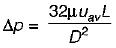...(i)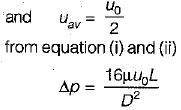QUESTION: 6

Laminar developed flow at an average velocity of 5 m/s occurs in a pipe of 10 cm radius. The velocity at 5 cm radius is

Solution: